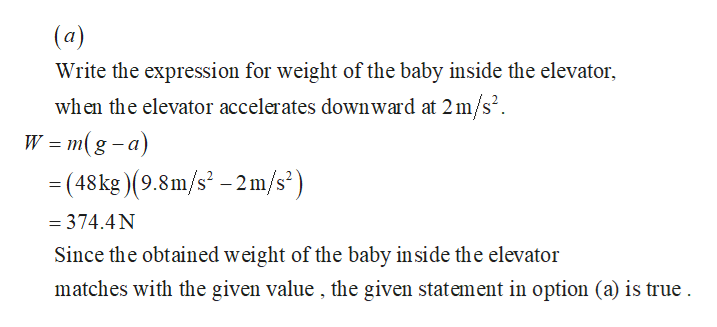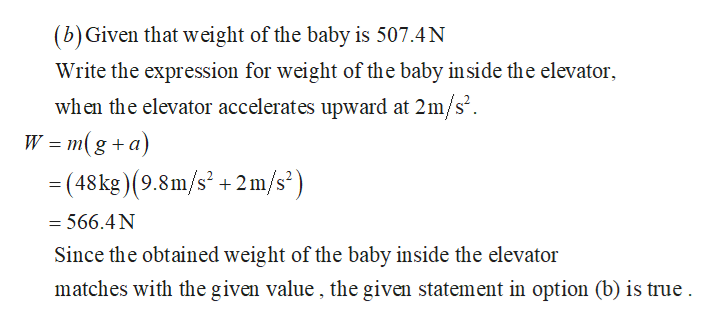# A child of mass 43 kg stands on a scale inside an elevator. Select one or more:A. The scale would show his weight as 335.40 N when the elevator accelerates downward at 2 m/s2.B. The scale would show his weight as 507.4 N when the elevator accelerates upward at 2 m/s2.C. The scale would show his weight as 335.40 N when the elevator accelerates upward at 2 m/s2.D. The scale would show his weight as 335.40 N when the elevator decelerates upward at 2 m/s2.E. The scale would show his weight as 421.4 N when the elevator is stationary.F. The scale would show his weight as 335.4 N when the elevator decelerates upward at 2 m/s2.G. The scale would show his weight as 421.40 N when the elevator moves with a constant velocity 2.5 m/s.

Question
70 views

A child of mass 43 kg stands on a scale inside an elevator. Select one or more:

A. The scale would show his weight as 335.40 N when the elevator accelerates downward at 2 m/s2.

B. The scale would show his weight as 507.4 N when the elevator accelerates upward at 2 m/s2.

C. The scale would show his weight as 335.40 N when the elevator accelerates upward at 2 m/s2.

D. The scale would show his weight as 335.40 N when the elevator decelerates upward at 2 m/s2.

E. The scale would show his weight as 421.4 N when the elevator is stationary.

F. The scale would show his weight as 335.4 N when the elevator decelerates upward at 2 m/s2.

G. The scale would show his weight as 421.40 N when the elevator moves with a constant velocity 2.5 m/s.

check_circle

Step 1

Given that a child of mass 43kg is inside an elevator.help_outlineImage Transcriptionclose(a) Write the expression for weight of the baby inside the elevator when the elevator accelerates downward at 2m/s2 W = m(g-a) = (48 kg ) (9.8m/s - 2m/s") = 374.4N Since the obtained weight of the baby inside the elevator matches with the given value , the given statement in option (a) is true fullscreen
Step 2help_outlineImage Transcriptionclose(b) Given that weight of the baby is 507.4N Write the expression for weight of the baby in side the elevator when the elevator accelerates upward at 2m/s2 W = m(g+a) - (48 kg)(9.8m/s2 +2m/s' = 566.4 N Since the obtained weight of the baby inside the elevator matches with the given value, the given statement in option (b) is true fullscreen

### Want to see the full answer?

See Solution

#### Want to see this answer and more?

Solutions are written by subject experts who are available 24/7. Questions are typically answered within 1 hour.*

See Solution
*Response times may vary by subject and question.
Tagged in

### Physics